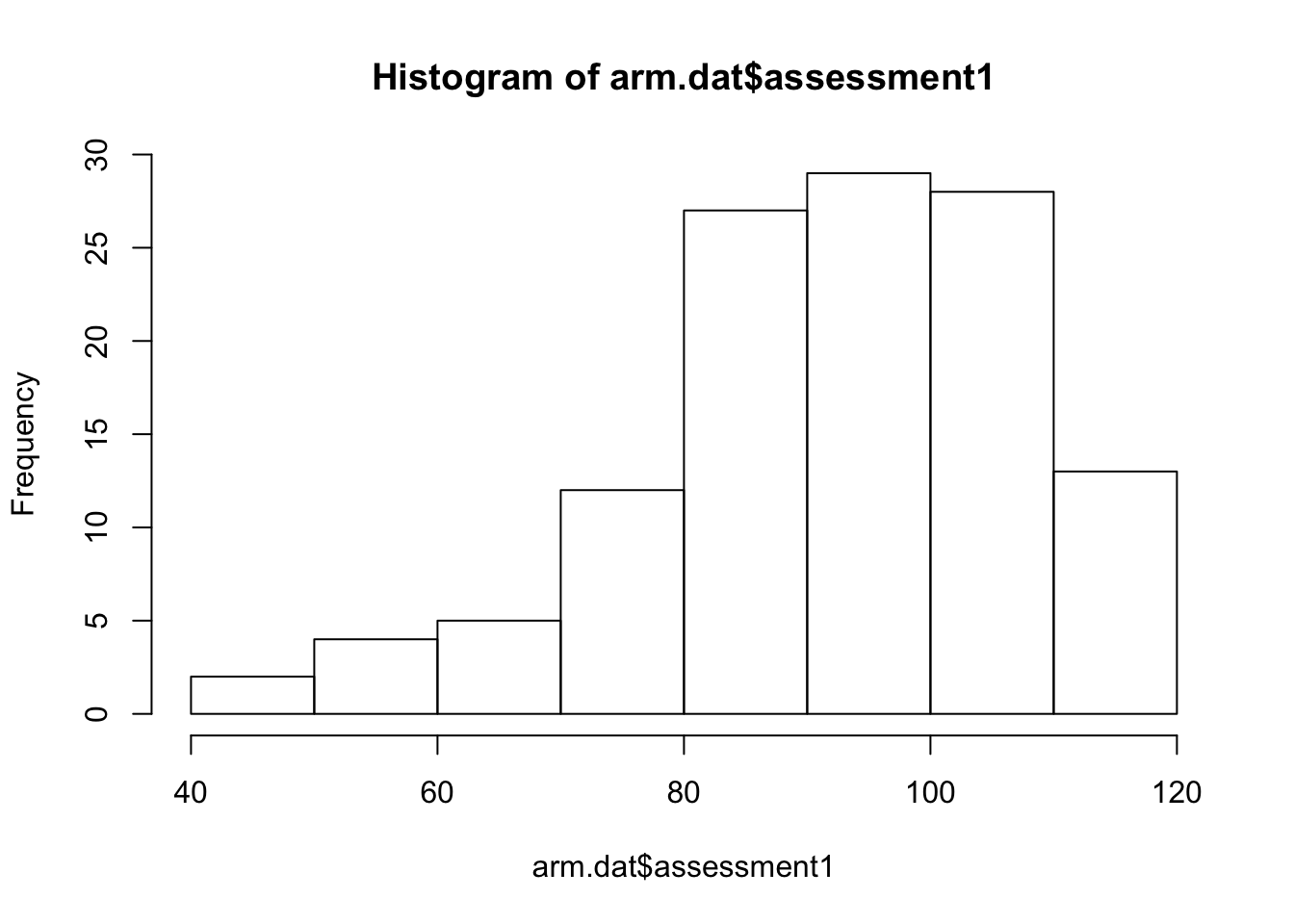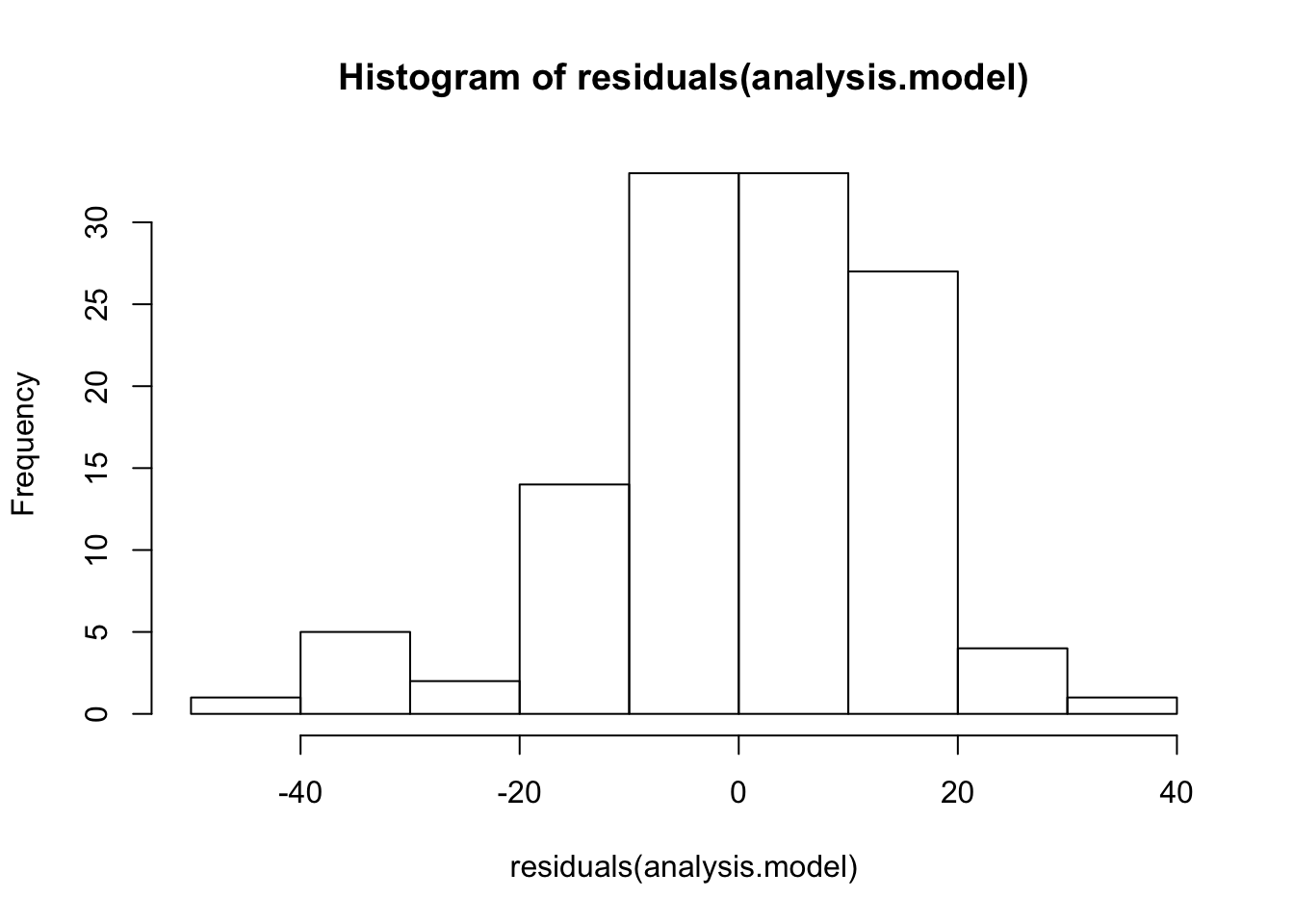# Introduction

When data fail to meet the assumptions of AOV - for example, when residuals are not normally distributed or treatment variances are not homogeneous, we may prefer to analysis the data using methods that do not depend on parametric assumptions. It is common in these cases to analyze the rank (ordered observations) instead of raw assessment values.

### Example Data

We will use winter wheat yield from the SDSU CPT trials, Brookings, SD, 2004. Frequently, yield dat is left-skewed (as shown in the histogram below), and common data transformations do not correct this type of skewness. Since assumptions of normality depend on symmetry in the distribution of the data, we might prefer a rank based analysis for this example.

hist(arm.dat$assessment1)analysis.model <- lm(assessment1 ~ treatment, data = arm.dat) For convenience, we can use the moments library to test for skewness and kurtosis of the residuals. hist(residuals(analysis.model))library(moments) agostino.test(residuals(analysis.model)) ## ## D'Agostino skewness test ## ## data: residuals(analysis.model) ## skew = -0.72938, z = -3.14090, p-value = 0.001684 ## alternative hypothesis: data have a skewness anscombe.test(residuals(analysis.model)) ## ## Anscombe-Glynn kurtosis test ## ## data: residuals(analysis.model) ## kurt = 3.6176, z = 1.4782, p-value = 0.1393 ## alternative hypothesis: kurtosis is not equal to 3 As we see, the assumption of normality of the residuals is not supported for the linear model. # Analysis of Ranks Analysis of ranks involves two steps. First, observations are assigned rank scores, usually from smallest to largest (the largest values given the largest ranks). Then, test statistics are calculated using rank scores. The most common are • Kruskal-Wallis (1952) • Friedmans (1937) • Align Rank Test (1990) ## Kruskal-Wallis Suppose we have a series of $$N$$ observations $$y_{ij}$$ that can be grouped into two or more groups ($$k=1 ... G$$). The Kruskal-Wallis analysis follows as 1. Assign a rank $$r_{ij} = \text{rank}(y_{ij})$$ tp all assessments, without regard to group. Typically, the smallest value is given the smallest rank (1). If values are identical (tied), then assign each assessments the average of the ranks over those values. 2. Calculate the test statistic ((Conover 1980))1 $T =\frac{1}{S^2} \left(\sum_{i=1}^{k} \frac{R^2_{i}}{n_i} - \frac{N (N-1)^2}{4}\right)$ where $$R_i = \sum_{j=1}^{n_i} r_{ij}$$ and $S^2 = \frac{1}{N-1} \left( \sum_{i} \sum_{k} r_{ij}^2 - N\frac{(N+1)^2}{4} \right)$ The test statistic $$T$$ can be compared to an exact $$T$$, but it is more common to approximate $$T$$ as $$\chi ^2$$ with $$g-1$$ d.f. When $$n_i$$ are small (~5) then exact tests should be preferred, but for large $$N$$ (~30) this becomes computationally intensive. An alternative notation comes from (Sprent and Smeeton 2001) $T = \frac{(N-1)(S_k-C)}{S_r - C}$ where $$S_r = \sum_{ij} r^2_{i,j}$$. We let $$s_i = \sum_{j=1}^{n_i}r_{ij}$$ and $$S_k = \sum_{i=1}^{k}\left(s_i^2 \ n_i \right)$$. This notation suggests the similarity to uncorrected total and treatment sums of squares. ### Multiple comparisons We can use treatment rank means ($$r_{i \cdot}$$) or treatment rank sums ($$R_{i \cdot}$$) to make comparisons among treatments. As with differences between means, we can write critical values as $\left| r_{i \cdot} - r_{j \cdot} \right| = \left| \frac{R_{i \cdot}}{n_i} - \frac{R_{j \cdot}}{n_j} \right| > t_{\nu,1-\alpha/2} \text{s.e.}_{rank}$ where $\text{s.e.}_{rank} = S \sqrt{ \frac{N-1-T}{N-k} }\sqrt{\frac{1}{n_i} + \frac{1}{n_j}}$ When we use Kruskal-Wallis tests, then $$\nu = N-k$$ 1. Assign Ranks arm.dat$rank <- rank(arm.dat$assessment1,ties.method='average') 1. Calculate rank sums and test statistic print(R <- tapply(arm.dat$rank, list(arm.dat$treatment), sum)) ## 1 2 3 4 5 6 7 8 9 10 11 12 13 14 15 16 17 18 ## 146 129 190 189 398 280 335 324 118 233 311 288 192 225 355 307 220 311 ## 19 20 21 22 23 24 25 26 27 28 29 30 ## 233 245 218 357 231 204 208 175 189 238 307 104 print(n <- tapply(arm.dat$rank, list(arm.dat$treatment), length)) ## 1 2 3 4 5 6 7 8 9 10 11 12 13 14 15 16 17 18 19 20 21 22 23 24 25 ## 4 4 4 4 4 4 4 4 4 4 4 4 4 4 4 4 4 4 4 4 4 4 4 4 4 ## 26 27 28 29 30 ## 4 4 4 4 4 print(k <- length(R)) ##  30 print(N <- sum(n)) ##  120 print(S2 <- (1/(N-1))*(sum(arm.dat$rank^2) - (N*(N+1)^2)/4))
##  1210
print(T <- (1/S2)*(sum(R^2/n)-(N*(N+1)^2)/4))
##  33.72066
1. Test against $$\chi^2$$
print(crit.chi <- qchisq(1 - alpha, df=k-1))
##  42.55697
print(1-pchisq(T,df=k-1))
##  0.2496326

Compare with the R function

kruskal.test(assessment1 ~ treatment, data=arm.dat)
##
##  Kruskal-Wallis rank sum test
##
## data:  assessment1 by treatment
## Kruskal-Wallis chi-squared = 33.721, df = 29, p-value = 0.2496

### Post hoc

means <- R/n
sd.rank <- sqrt(S2*((N-1-T)/(N-k)))
post.tbl <- matrix(0,nrow=k,ncol=k)
crit.table <- matrix(0,nrow=k,ncol=k)
crit.t <- qt(1-alpha/2,N-k)
for(i in 1:(k-1)) {
for(j in (i+1):k) {
post.tbl[i,j] <- abs(means[i]-means[j])
crit.table [i,j] <- crit.t*sd.rank*(sqrt(1/n[i]+1/n[j]))
post.tbl[j,i] <- post.tbl[i,j]>crit.table[i,j]
crit.table [j,i] <- 2*(1-pt(post.tbl[i,j]/(sd.rank*(sqrt(1/n[i]+1/n[j]))),N-k))
}
}
post.tbl[1:5,1:5]
##      [,1] [,2]  [,3]  [,4]  [,5]
## [1,]    0 4.25 11.00 10.75 63.00
## [2,]    0 0.00 15.25 15.00 67.25
## [3,]    0 0.00  0.00  0.25 52.00
## [4,]    0 0.00  0.00  0.00 52.25
## [5,]    1 1.00  1.00  1.00  0.00
crit.table[1:5,1:5]
##            [,1]         [,2]        [,3]        [,4]     [,5]
## [1,] 0.00000000 47.566924368 47.56692437 47.56692437 47.56692
## [2,] 0.85951063  0.000000000 47.56692437 47.56692437 47.56692
## [3,] 0.64703701  0.525787456  0.00000000 47.56692437 47.56692
## [4,] 0.65452302  0.532580604  0.99169217  0.00000000 47.56692
## [5,] 0.01000859  0.006099384  0.03249823  0.03169403  0.00000

### Other examples

To analyze ranks in ARM, use the AR (auto rank) code.

#### Kruskal 2.1

These data reproduce Table 2.1 from (1952). Column two is a duplicate of column one, but column two contains the AR action code, while one does not.

## Friedmans

Friedman’s analysis assigns ranks within whole blocks, and is only applicable when blocks are balanced (all treatments appear in each block). When ties are present, we write ((Sprent and Smeeton 2001), p 211). If the are missing assessments, or when there higher-order blocking (i.e. Latin squares) is present, we use the Aligned Rank Test.

$S_r = \sum _{i,j} r^2_i$

where $$r_{i j}$$ is the rank assigned treatment $$i$$ in block $$j$$. We let

$S_k = \frac{\sum _{i=1} ^k s^2_i}{b}$

where $$s_i = \sum_{j=1} ^k r_{ij}$$ We also calculate a correction factor

$C = \frac{b t (t+1)^2}{4}$ We then calculate the test statistic

$T = \frac{b (t-1) (S_t - C)}{S_r - C}$

#assume we've excluded all missing values
UniqueTreatments <- unique(arm.dat$treatment) UniqueReplicates <- unique(arm.dat$replicate)
for(rep in UniqueReplicates) {
mask <- arm.dat$replicate == rep arm.dat$rank[mask] <- rank(arm.dat$assessment1[mask], ties.method='average') } s <- tapply(arm.dat$rank, list(arm.dat$treatment), sum) b <- length(UniqueReplicates) k <- length(UniqueTreatments) S_r <- sum(arm.dat$rank^2)
S_k <- sum(s^2)/b
C <- (b*k*(k+1)^2)/4
print(T <- (b*(k-1)*(S_k - C))/(S_r-C))
##  52.72258

When $$b$$ and $$k$$ are not too small, then $$T$$ can be approximated as $$\chi ^2$$ with $$k-1$$ d.f.

print(1-pchisq(T,df=k-1))
##  0.004523279
print(qchisq(1 - alpha, df=k-1))
##  42.55697

We can compare this to the built-in R function,

friedman.test(assessment1 ~ treatment | replicate, data = arm.dat)
##
##  Friedman rank sum test
##
## data:  assessment1 and treatment and replicate
## Friedman chi-squared = 52.723, df = 29, p-value = 0.004523

This function will fail if all observations are missing for any single treatment (but this can be corrected by reordering treatments as factors).

In the cases where there are no ties, $$S_r = b k (k+1)(2k+1)$$ and $$T$$ simplifies to

$T = \frac{12 \sum _{i=1} ^t s^2_i}{bt(t-1)} - 3b(t+1)$

print(T <- (12*sum(s^2))/(b*k*(k+1)) - 3*b*(k+1))
##  52.72258

### Post hoc

For treatment comparisons, we calculate a minimum difference of rank sums by

$\left| R_{i \cdot} - R_{j \cdot} \right| > t_{\nu,1-\alpha/2} \text{s.e.}_{R}$

where $\text{s.e.}_{R} = \sqrt{ \frac{2 b}{(b-1)(k-1)} }\sqrt{S_r - S_k}$

err.df <- (k-1)
crit.t <-qt(1 - 0.05 / 2, err.df)

A <- sum(arm.datrank^2) A2 <- (b*k*(k+1)*(2*k+1))/6 B <- sum(s^2)/b T <- ((b-1)*(B-(b*k*(k+1)^2)/4))/(A-B) print(sqrt((2*b*(A-B))/((b-1)*(k-1)))/b) ##  5.308852 ### Other examples #### Friedman Table 1 These data reproduce Table 1 from (1937). There are three columns in the data, all identical. The first is marked IID, while the third is marked AR. The second column is AS transformed; this is suggested by the nature of the assessment (standard deviations of expenditures at different income levels) ## Aligned Ranks Lehmann and D’Abrera (1975) proposed’aligning’ observations by subtracting some estimate of block effect from each observation that block, then performing analysis of ranks on the aligned values. Consider the block-only model $y_{ij} = \mu + b_{j} + \epsilon_{ij}$ We calculate $$\epsilon'_{ij} = y_{ij} - (\mu +\widehat{b}_{j})$$ and then rank $r'_{ij} = \text{rank}(\epsilon'_{ij})$ See - ARM Trial analysis.model <- lm(assessment1 ~ treatment, data = arm.dat) block.lm <- lm(assessment1 ~ replicate, data = arm.dat) arm.datAlignedRank  <- rank(resid(block.lm))[row.names(arm.dat)]
#In most cases, we want rank to be sch that the smallest values have the lowest rank. This preserves the
#relative order of treatment means.
arm.dat$AlignedRank <- max(arm.dat$AlignedRank,na.rm=TRUE) - arm.dat\$AlignedRank + 1
rank.test <- kruskal.test(AlignedRank ~ treatment, arm.dat)
rank.test
##
##  Kruskal-Wallis rank sum test
##
## data:  AlignedRank by treatment
## Kruskal-Wallis chi-squared = 53.852, df = 29, p-value = 0.003365

# Latin Square

Because Latin squares are blocked in two dimensions, both Kruskal-Wallis and Friedman’s test can be expected to be much less powerful, compared to the appropriate parametric analysis. In this case, we prefer to use the aligned rank method (Kruskal-Wallis on the residuals of the block model).

Best and Rayner (2011) compared four methods of the analysis of ranks for a Latin square design. We reproduce these in part in:

1. This is a simplification of the formula from (Kruskal 1952), used when ties are present